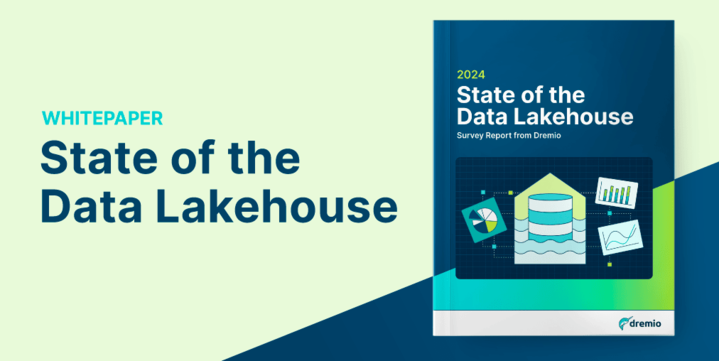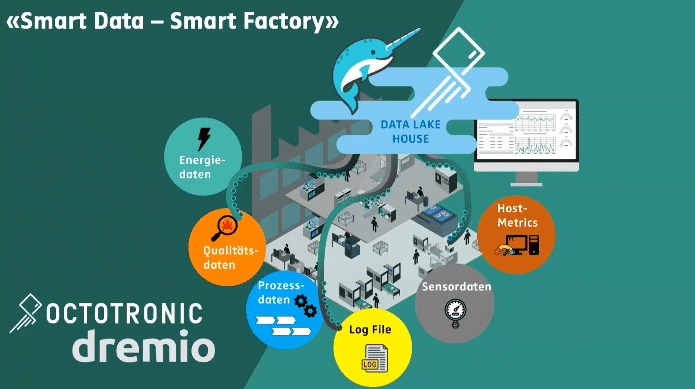# Data Lake Machine Learning Models with Python and Dremio

## Introduction

Amazon Simple Storage Service (S3) is an object storage service that offers high availability and reliability, easy scaling, security, and performance. Many companies all around the world use Amazon S3 to store and protect their data.

PostgreSQL is an open-source object-relational database system. In addition to many useful features, PostgreSQL is highly extensible, and this allows to organize work with the most complicated data workloads easily.

In this article, we will show how to load data into Amazon S3 and PostgreSQL, then how to connect these sources to Dremio, and how to perform data curation. After that, we will use Python to solve one of the main tasks of machine learning – classification with supervised machine learning algorithms.

We will use HTRU2 dataset which describes a sample of pulsar candidates collected during the High Time Resolution Universe Survey. As machine learning models for classification of pulsar signals from the noise, we’ll use the Gradient boosting algorithms, XGBoost and lightGBM.

## Assumptions

In this tutorial, we assume that you have the following items already installed and setup:

## Data overview

HTRU2 dataset describes a number of features of the pulsar candidates. These features are presented as numerical data received from radio signals of telescopes. Almost all detections (90%) for pulsar “candidate” are caused by radio frequency interference and noise, making real signals hard to find.

The features are listed below:

1. Mean of the integrated profile.
2. Standard deviation of the integrated profile.
3. Excess kurtosis of the integrated profile.
4. Skewness of the integrated profile.
5. Mean of the DM-SNR curve.
6. Standard deviation of the DM-SNR curve.
7. Excess kurtosis of the DM-SNR curve.
8. Skewness of the DM-SNR curve.
9. Class.

There are 17,898 total examples, 1,639 positive and 16,259 negative.

We will split the data by “Integrated pulse” and “DM-SNR curve” features and make a common “id” column for correct data merging. After that, we will load the data respectively into Amazon S3 and PostgreSQL storages.

We will start by loading data into Amazon S3 storage. After registering for the service, create a bucket by clicking on the Create bucket button.

If you need to set access permissions or other properties for the bucket – click on the Next button and select the necessary parameters.

As in the previous case for the bucket, if you need to set some specific options for the file – click on the Next button and select them. Once you have uploaded a file, you can view its properties, access, URL-link, and content just by clicking on it.

To access the uploaded file in Dremio, you will need security parameters, namely Access key ID and Secret access key.

To get them, click on IAM in the Security, Identity, & Compliance section in the list of services in AWS Console:

Then select the user.

And select security settings.

Finally, you can get the necessary security parameters.

Now, we have everything we need to access our Amazon S3 data from Dremio.

Next, let’s upload the second part of the dataset to PostgreSQL database.

First, you need to install a server and utilities that match your operating system. In our case, we will choose the 11 version of PostgreSQL for Windows platform and pgAdmin 4 for managing the server. Installation is simple and takes a few minutes. The only thing to note is that port 5432 (default port) should be free.

After the installation is finished, let’s start pgAdmin and connect to the PostgreSQL server. You need to create your own server as shown on the screenshot below.

The next step is to create a database

Then, we’ll use the official tutorial for uploading data to the PostgreSQL database and create the database table for uploading the second part of HTRU2’s dataset.

As a result, we got the following data table.

## Connecting Dremio to Amazon S3 and PostgreSQL

In the Sources section, click on the corresponding button to add a new source and select Amazon S3 database. In the General tab:

• Set a name for your source
• Enter the AWS Access Key
• Click the Save button.

And similar steps are required to connect the PostgreSQL database. Choose PostgreSQL from the data sources and fill in the parameters as shown on the screenshot below:

After that, click on the dataset and specify the next parameters:

• Data format – Text (delimited)
• Field delimiter – Comma
• Extract Field Names – for saving columns names.

Then perform the same steps for the second database.

## Data curation in Dremio

First, we open one of the datasets and save it in our previously created Dremio-space.

Next, join the datasets into one by clicking the Join button.

The key column for datasets merging is the ID column.

Note that the values of ID columns should be in integer format.

After that, we can drop the ID columns as we don’t need them anymore.

Next, we need to set the appropriate data types for our columns, namely:

• for all features – set float data format
• for target class – set integer data format.

It is possible to export data from Dremio in the CSV file. It can be convenient for fast-prototyping. But we’ll use direct connecting to Dremio-server for data loading.

## Building machine learning models

Machine learning models are usually used to construct data for one of the following tasks:

• Classification
• Clustering
• Predictions
• Ranking
• Detection and recognition
• Analytical structures.

For our Pulsar Detection task, will use machine learning classifiers. Among the classic ML classifiers, we want to highlight the following models:

• Logistic Regression
• SVM
• Naive Bayes
• KNN
• Decision Tree
• Random Forest.

We are going to use simple and effective methods based on Gradient boosting algorithms. Gradient boosting is usually used to solve regression and classification tasks. These models are usually an ensemble of weak prediction models (decision trees).

There are a lot of Gradients boosting models, but in this article, we will use 2 popular models, XGBoost and LightGBM.

To connect Dremio with Python, we will use the ODBC driver. Also, you need to have pyodbc Python package installed. If everything is installed properly, the connection becomes easy.

```import pandas as pd
import pyodbc
import os
from glob import glob
## Only for exported CSV-file
## Path to data
# csv_file = os.path.abspath(os.curdir)\
#            	+ '\\htru-2-pulsar-star-data-htru-2-dataset.csv'
# From Dremio
# Setup the ODBC connection to the Dremio instance
user_id = 'user'
cnxn = pyodbc.connect(r"DSN=Dremio Connector;UID={user};PWD={pwd}"
.format(user=user_id,

# SQL style query to retrieve the required data from Dremio
sql = r'SELECT * FROM "HTRU 2 - Pulsar Star Data"."HTRU2-Dataset"'
# Executing the query using the already open ODBC connection

To perform correct data analysis, it is necessary to separate features and target class columns.

```# target class
y = df.targetclass
# features
x = df.drop(['targetclass'], axis = 1)```

There were no null-values in the description of the dataset, but to make sure that the ML model will work properly, it is necessary to double-check that they are missing.

`print(x.isnull().sum())`

Null-values in the dataset are missing, so we can move to the next step. We will build a correlation matrix to detect the correlation between the features.

```import matplotlib.pyplot as plt
import seaborn as sns
plt.figure(figsize=(8,6))
sns.heatmap(x.corr(), annot=True, linewidth=2, cmap=plt.cm.Blues)
plt.title('Corellation of Features \n', weight='bold', fontsize=16)
plt.xticks(rotation=45)
plt.show()```

As you can see, the features are weakly dependent on each other, and it is impossible to ignore any of them.

Data description notes that the number of the target class is equal to two: negative 0 and positive 1. Let’s double check this and at the same time count them.

```# target count
count_data = df['targetclass'].groupby(df['targetclass']).count()
print('type: ', count_data)
# plot
plt.figure(figsize=(3, 6))
ax = sns.countplot(x='targetclass',
palette=sns.color_palette("RdBu_r", 7),
data=df);
for p in ax.patches:
height = p.get_height()
ax.text(p.get_x() + p.get_width()/2., height + 200,
f'{height/len(y)* 100:1.1f} %', ha="center")
plt.grid(ls = "--", zorder = 1)
plt.title('Counting Data \n', weight='bold', fontsize=16)
plt.show()```

The next step is Data processing. To do this, we will rescale the data and split it into categories: train, valid, and test in the proportion of 70%, 18%, and 12%.

```from sklearn.preprocessing import StandardScaler
#Scaling
sc = StandardScaler()
X = sc.fit_transform(x)
from sklearn.model_selection import train_test_split
# Split data to train, valid test data
# Proportion 70 %  // 18 %  // 12 %
X_train, X_val_test, y_train, y_val_test = train_test_split(X, y, test_size=0.7, random_state=1)
X_val, X_test, y_val, y_test = train_test_split(X_val_test, y_val_test, test_size=0.4, random_state=1)```

In our case, we don’t try to achieve the highest score, so we will drop data augmentation and cross-validation steps.

Now we are ready to build machine learning classifiers.

### LightGBM

LightGBM is a gradient boosting framework that uses decision trees learning algorithms.

Most ML classifiers that use gradient boosting algorithms have common and identical parameters:

• n_estimators – the number of boosted decision trees to fit
• learning_rate – boosting the learning rate
• early_stopping_rounds – parameter that helps to stop the model’s overfitting.

We’ll select approximately the same value of these parameters for this model and for the next one.

Let’s create a LightGBM Classifier model.

```import lightgbm as lgb
classifier_lgb = lgb.LGBMClassifier(n_estimators=1000, learning_rate=0.01)
classifier_lgb.fit(X_train, y_train, eval_set=[(X_train, y_train), (X_val, y_val)], early_stopping_rounds=50)```

The result of the model training is shown below.

Let’s check the results by comparing them with the test data.

```from sklearn.metrics import accuracy_score,f1_score, precision_score, recall_score
y_pred_lgb = classifier_lgb.predict(X_test)
train_score_lgb = classifier_lgb.score(X_train, y_train)
test_score_lgb = classifier_lgb.score(X_test, y_test)
from sklearn.metrics import classification_report
print(classification_report(y_test, y_pred_lgb))```

The result shows that our model is not overfitted and gets more than 0.97 score.

Let’s also look at the Confusion matrix demonstrating the quality of the classifier’s work.

```from sklearn.metrics import confusion_matrix
cm_lgb = confusion_matrix(y_test, y_pred_lgb)
#plot
plt.figure(figsize=(6, 4))
plot_confusion_matrix(cm_lgb, classes=list(count_data.index),
title='Confusion matrix, LightGBM')
plt.show()```

The confusion matrix confirms the good quality of our LightGBM classifier.

### XGBoost

XGBoost is a gradient boosting method that also uses decision trees learning algorithms.

XGBoost classifier model can be created as shown below.

```import xgboost as xgb
classifier_xgb = xgb.XGBClassifier(max_depth=3, n_estimators=1000, learning_rate=0.05)
classifier_xgb.fit(X_train, y_train, eval_set=[(X_train, y_train), (X_val, y_val)],
early_stopping_rounds=50)```

After model training, we got the following results.

A comparison with the test data gives the following scores.

This model, just like the previous one, hasn’t been overfitted and gets approximately the same scores. The confusion matrix for the resulting XGBoost classifier is shown below.

We can conclude that the result is good, which is generally the same as for the previous model.

To improve the results of the ML models, you can perform the following steps:

• Increase features by analyzing the importance of current features.
• Use hyperparameter selection for ML models.
• Select a more accurate model (or use models ensemble).
• Use cross-validation and data augmentation.

## Conclusion

In this article, we described how to create machine learning models for the gradient boosting classifiers, LightGBM Boost and XGBoost, using Amazon S3 and PostgreSQL databases and Dremio. Dremio helped us to work with different databases and combine all the data in one dataset.

## Ready to Get Started? Here Are Some Resources to Help### 2024 State of the Data Lakehouse

Benchmark your organization with Dremio's State of the Data Lakehouse Survey Report!### Expert Panel Discussion – Data Integration Trends and Best Practices Webinar

TDWI senior research director James Kobielus will engage data industry experts in an in-depth discussion of data integration trends and best practices### Smart Data – Smart Factory with Octotronic and Dremio

Wie bringt ein Data Lake House die Smart Factory auf ein neues Level?